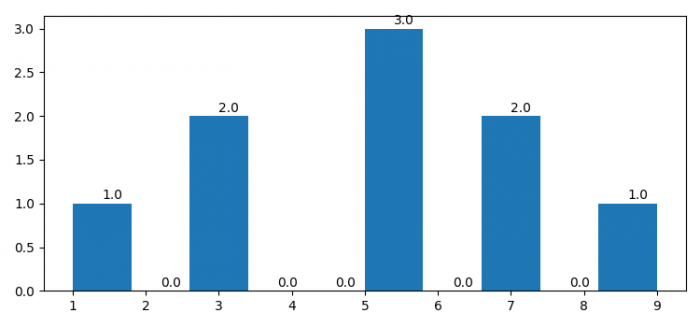# How to display the count over the bar in Matplotlib histogram?

MatplotlibPythonData Visualization

To display the count over the bar in matplotlib histogram, we can iterate each patch and use text() method to place the values over the patches.

## Steps

• Set the figure size and adjust the padding between and around the subplots.
• Make a list of numbers to make a histogram plot.
• Use hist() method to make histograms.
• Iterate the patches and calculate the mid-values of each patch and height of the patch to place a text.
• To display the figure, use show() method.

## Example

import matplotlib.pyplot as plt

plt.rcParams["figure.figsize"] = [7.50, 3.50]
plt.rcParams["figure.autolayout"] = True

data = [3, 5, 1, 7, 9, 5, 3, 7, 5]
_, _, patches = plt.hist(data, align="mid")

for pp in patches:
x = (pp._x0 + pp._x1)/2
y = pp._y1 + 0.05
plt.text(x, y, pp._y1)

plt.show()

## Output